## The scale space

Series: SIFT: Theory and Practice:

Real world objects are meaningful only at a certain scale. You might see a sugar cube perfectly on a table. But if looking at the entire milky way, then it simply does not exist. This multi-scale nature of objects is quite common in nature. And a scale space attempts to replicate this concept on digital images.

## Scale spaces

Do you want to look at a leaf or the entire tree? If it's a tree, get rid of some detail from the image (like the leaves, twigs, etc) intentionally.

While getting rid of these details, you must ensure that you do not introduce new false details. The only way to do that is with the Gaussian Blur (it was proved mathematically, under several reasonable assumptions).

So to create a scale space, you take the original image and generate progressively blurred out images. Here's an example: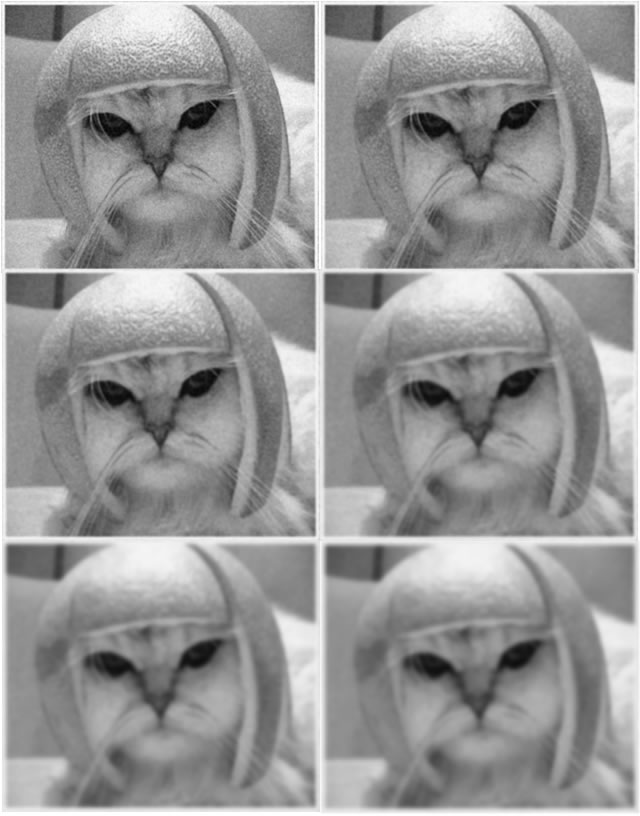Look at how the cat's helmet loses detail. So do it's whiskers.

## Scale spaces in SIFT

SIFT takes scale spaces to the next level. You take the original image, and generate progressively blurred out images. Then, you resize the original image to half size. And you generate blurred out images again. And you keep repeating.

Here's what it would look like in SIFT:Images of the same size (vertical) form an octave. Above are four octaves. Each octave has 5 images. The individual images are formed because of the increasing "scale" (the amount of blur).

## The technical details

Now that you know things the intuitive way, I'll get into a few technical details.

Octaves and Scales

The number of octaves and scale depends on the size of the original image. While programming SIFT, you'll have to decide for yourself how many octaves and scales you want. However, the creator of SIFT suggests that 4 octaves and 5 blur levels are ideal for the algorithm.

The first octave

If the original image is doubled in size and antialiased a bit (by blurring it) then the algorithm produces more four times more keypoints. The more the keypoints, the better!

Blurring

Mathematically, "blurring" is referred to as the convolution of the gaussian operator and the image. Gaussian blur has a particular expression or "operator" that is applied to each pixel. What results is the blurred image.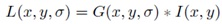The symbols:

• L is a blurred image
• G is the Gaussian Blur operator
• I is an image
• x,y are the location coordinates
• σ is the "scale" parameter. Think of it as the amount of blur. Greater the value, greater the blur.
• The * is the convolution operation in x and y. It "applies" gaussian blur G onto the image I.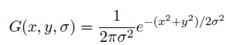This is the actual Gaussian Blur operator.

Amount of blurring

The amount of blurring in each image is important. It goes like this. Assume the amount of blur in a particular image is σ. Then, the amount of blur in the next image will be k*σ. Here k is whatever constant you choose.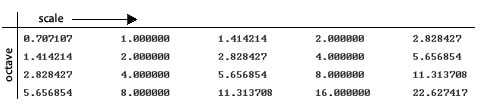This is a table of σ's for my current example. See how each σ differs by a factor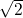from the previous one.

## Summary

In the first step of SIFT, you generate several octaves of the original image. Each octave's image size is half the previous one. Within an octave, images are progressively blurred using the Gaussian Blur operator.

In the next step, we'll use all these octaves to generate Difference of Gaussian images.

## More in the series

This tutorial is part of a series called SIFT: Theory and Practice:

Utkarsh Sinha created AI Shack in 2010 and has since been working on computer vision and related fields. He is currently at Microsoft working on computer vision.